Many systems are designed to include an arbitrary number of similar or identical components. A good example of such a system is the Internet, which is designed to accommodate a large number of hosts, all communicating using the same set of protocols. Another example would be peer-to-peer protocols such as Chord.

We call these parameterized systems where the parameter in question is the number of protocol participants. Ivy allows you to model an implement parameterized protocol in a particular style. A parameterized object is one in which every component has an initial parameter of the same type. Here is an example of an object parameterized on type `t`:

``````type t

object foo = {

function bit(S:t) : bool
after init {
bit(S) := false
}

action set_bit(self:t) = {
bit(self) := true
}
}
``````

Notice that both the state component `bit` and the action `set_bit` have a first parameter of type `t`. The parameter of `set_bit` is suggestively called `self`. This parameter is used in any references to state components within the action. Thus, object `foo` really acts like a collection of independent objects or processes, one for each element of type `t`. The type `t` acts like a reference to one of these objects.

IVy provides a shorthand for parameterized objects. We can equivalently write the object `foo` as follows:

``````type t

object foo(self:t) = {

individual bit : bool
after init {
bit := false
}

action set_bit = {
bit := true
}
}
``````

Ivy adds the parameter `self` to each state component of `foo`, and each reference to a component. That is, `self` becomes an implicit parameter, much as it does in an object-oriented programming language (except for Python, where the `self` parameter is explicit). It makes no difference to IVy whether you use implicit or explicit parameters. You can reason about IVy programs in the same way using either style.

As we will see later, IVY has special support for parameterized objects. For example, you can compile them and run them in separate process spaces or on different hosts. In addition, when proving assertions that relate to only one process, you can ignore the parameter. This can be a good trick for staying within a decidable logical fragment.

As an example of a parameterized protocol, lets look at the very simple leader election protocol, introduced in this paper in 1979.

In this protocol we have a collection of distributed processes organized in a ring. Each process can send messages to its right neighbor in the ring and receive message from left neighbor. A process has a unique `id` drawn from some totally ordered set (say, the integers). The purpose of the protocol is to discover which process has the highest `id` value. This process is elected as the “leader”.

This protocol itself is trivially simple. Each process transmits its own `id` value. When it receives a value, it retransmits the value, but only if it is greater than the process’ own `id` value. If a process receives its own `id`, this value must have traveled all the way around the ring, so the process knows its `id` is greater than all others and it declares itself leader.

``````object asgn = {

function pid(X:node.t) : id.t          # map each node to an id

axiom [injectivity] pid(X) = pid(Y) -> X = Y
}

object serv = {

action elect(v:node.t)                 # called when v is elected leader

object spec = {                        # only the max pid can be elected
before elect {
assert asgn.pid(v) >= asgn.pid(X)
}
}
}
``````

These two objects are parameterized on an abstract datatype `node` that we will define shortly. Type `node.t` represents a reference to a process in our system. The `asgn` object defines an assignment of `id` values to nodes, represented by the function `pid`. The fact that the `id` values are unique is guaranteed by the axiom `injectivity`.

The service specification `serv` defines one action `elect` which is called when a given node is elected leader. Its specification says that only the node with the maximum `id` value can be elected.

Now that we know what the protocol is supposed to do, let’s define the protocol itself. First, we use the explicit parameter style:

``````object app = {

action async(me:node.t) = {
call trans.send(node.get_next(me),asgn.pid(me))
}

implement trans.recv(me:node.t,v:id.t) {
if v = asgn.pid(me) {       # Found a leader!
call serv.elect(me)
}
else if v > asgn.pid(me)  { # pass message to next node
call trans.send(node.get_next(me),v)
}
}

}
``````

Our protocol implementation refers to two interfaces: the `serv` interface we just defined, and a network transport interface `trans` defined below. It also refers to the abstract datatype `node` that we will also define shortly.

The protocol provides an action `async` that, when called, transmits the node’s `id` to the next process in the ring, defined by the the action `node.get_next` (here, we used `me` instead of `self` to save two characters).

The protocol also implements an action of the transport interface `trans.recv`. This is called when the process receives a message with an `id` value `v`. If the value is equal to the process’ own `id`, the process knows it is leader and calls `serv.elect`. Otherwise, if the received value is greater, it calls `trans.send` to send the value on to the next node.

Notice that when giving the implementation, we explicitly gave the parameters of `trans.recv` rather than inheriting them from the interface specification. This can avoid confusion and also allows us to give whatever local names we want to the parameters.

Here is the same object described in the implicit style:

``````object app(me:node.t) = {

action async = {
call trans.send(node.get_next(me),asgn.pid(me))
}

implement trans.recv(v:id.t) {
if v = asgn.pid(me) {       # Found a leader!
call serv.elect(me)
}
else if v > asgn.pid(me)  { # pass message to next node
call trans.send(node.get_next(me),v)
}
}

}
``````

There is not much difference. Notice that we dropped the parameter `me` from the action definition. However, references to other objects still have to have the explicit parameter `me`. The implicit style mainly shows an advantage when the parameterized object has many references to its own actions and state components.

With our protocol implemented, let’s look at the interfaces that it’s built on, starting with the type of `id` values:

``````module totally_ordered(t) = {
relation (X:t < Y:t)
property [transitivity] X:t < Y & Y < Z -> X < Z
property [antisymmetry] ~(X:t < Y & Y < X)
property [totality] X:t < Y | X = Y | Y < X
}

module total_order = {
type t
instantiate totally_ordered(t)   # t is totally ordered
}

instance id : total_order
``````

Notice that we defined a couple of modules that we can re-use later. The first gives the axioms of a total order. The second defines a datatype that obeys these axioms. We then define our abstract type `id` to be an instance of `total_order`. Later, we will implement `id.t` with fixed-width integers.

Now let’s look at the type of node references, which is a bit more interesting:

``````module ring_topology = {
type t
instantiate total_order_axioms(t)   # t is totally ordered

action get_next(x:t) returns (y:t)

object spec = {
after get_next {
assert (x < y & ~ (x < Z & Z < y)) | (y <= X & X <= x)
}
}
}

instance node : ring_topology
``````

We start by defining a generic module for types that are organized in a ring topology. We use a totally ordered type `t` for the ring elements. In addition, the `get_next` action takes an element `x` and returns the next element `y` in the ring. The specification says that either `y` is the least element larger than `x` or `x` is the maximum element and `y` the minimum (that is, we “wrap around” to the beginning). In principle, we should also say that the set `t` is finite, but we won’t actually need this fact to prove safety of our protocol. This is one way of specifying a ring topology. Later we will see a different way that can make the proofs a little simpler. We can implement either version with fixed-width integers.

Finally, we need the service specification for the network transport layer. It’s quite simple:

``````object trans = {

relation sent(V:id.t, N:node.t)
after init {
sent(V, N) := false
}

action send(dst:node.t, v:id.t)
action recv(dst:node.t, v:id.t)

object spec = {
before send {
sent(v,dst) := true
}
before recv {
assert sent(v,dst)
}
}
}
``````

The relation `sent` tells us which values have been sent to which nodes. Initially, nothing has been sent. The interface provides two actions `send` and `recv` which are called, respectively, when a value is sent or received. The `dst` parameter gives the destination node of the message.

The specification says that a value is marked as sent when `send` occurs and a value must be marked sent before it can be received. This describes a network service that can duplicate or re-order messages, but cannot corrupt messages.

## Verifying the leader election protocol

Now let’s try to verify that our leader election protocol `app` guarantees its service specification `serv` , assuming the specifications of `trans`, `node`, `id` and `asgn`. Here is the isolate declaration:

``````isolate iso_p = app with node,id,trans,serv,asgn
``````

We are trying to prove that, when any node calls `serv.elect`, it in fact has the highest `id`. That is, the `before` specification of `serv.elect` is a guarantee of `app` when it calls `serv.elect`.

To get a sense of what we’re proving, let’s have a look at the isolate:

``````\$ ivy_show isolate=iso_app leader_election_ring.ivy

... various declarations ...

action trans.send(dst:node.t,v:id.t) = {
trans.sent(v,dst) := true
}

action ext:trans.recv(dst:node.t,v:id.t) = {
assume trans.sent(v,dst);
if v = asgn.pid(dst) {
call serv.elect(dst)
}
else {
if v > asgn.pid(dst) {
local loc {
call loc := node.get_next(dst);
call trans.send(loc, v)
}
}
}
}

action serv.elect(v:node.t) = {
assert asgn.pid(v) >= asgn.pid(X)
}

action ext:app.send(me:node.t) = {
local loc {
call loc := node.get_next(me);
call trans.send(loc, asgn.pid(me))
}
}

action node.get_next(x:node.t) returns(y:node.t) = {
assume ((x < y & ~(Z:node.t < y & x < Z)) | (x = node.tail & y = node.head))
}
``````

Notice that the assertion in `serv.elect` is preserved as a guarantee. However, the other assertions have become assumptions.

Obviously, we will need an inductive invariant at this point. We will try to discover one using IVy’s CTI method. We start IVy using this command:

``````\$ ivy ui=cti isolate=iso_app leader_election_ring.ivy
``````

We start, as usual, with `Invariant|Check induction`. Ivy tells us the the safety check failed, meaning that an assert fails in some action: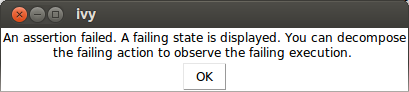When we click OK, this is what we see: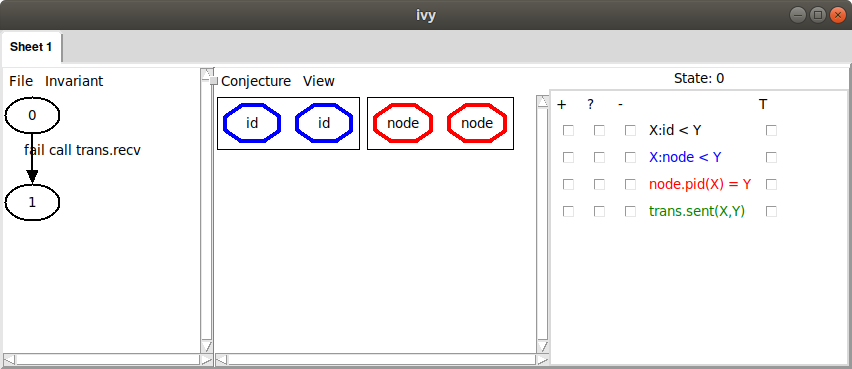On the left, we see a failing transition starting in state `0`, using one of our two exported actions, `app.async` and `trans.recv`. To discover which action failed, and explore the execution path to the failure, we can left-click on the transition and choose `Decompose`. In this case, however, we already have a pretty good idea of what went wrong: some node must have received its own `id` and declared itself leader without having the highest `id`. This is not surprising, since with no invariant conjectures, the only thing we know about state `0` is that it satisfies our axioms.

On the right, we see a depiction of the state `0`. There are two elements in each of the two sorts `id.t` and `node.t`. Since we haven’t yet selected any relations to view, this is all we see. We could start selecting relations to see more information, but let’s instead choose the command `Invariant|Diagram` to see what information IVy thinks might be relevant to the failure. Here’s what we see: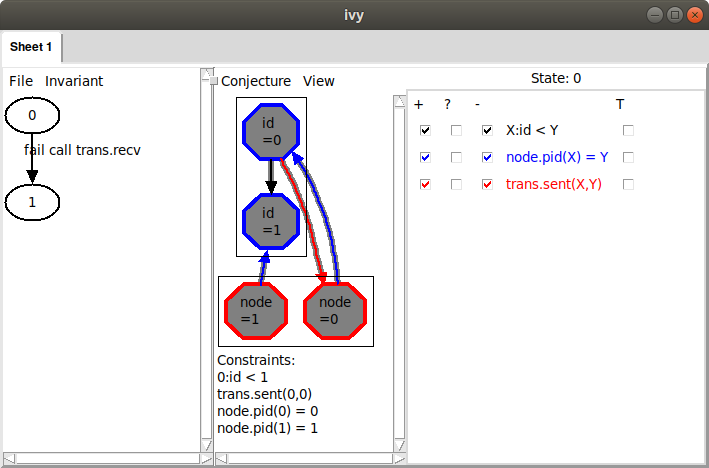The arrow from id 0 to id 1 is depicting the relation `X:id.t < Y`. Because the arrow goes from `X` to `Y`, we interpret the arrow to mean `0:id.t < 1`. Similarly, the arrow from node 0 to id 0 means that `asgn.pid(0) = 0`. That is, the id of node 0 is 0. We can also see that node 1’s id is 1 and that `trans.sent(0,1)`, in other words, id 0 has been sent to node 0. These facts are displayed below the graph under the heading “Constraints”.

The problem is clear: node 1 is receiving its own id, so it is about to become leader, but it does not have highest id. This situation clearly has to be ruled out. We do this by selecting the `Conjecture|Strengthen` command. Here is what IVy says: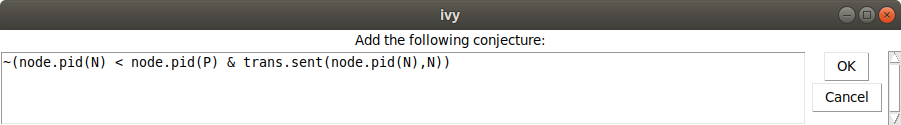In other words, we conjecture that it never happens that a node N has id less than a node P, and node N is receiving its own id. Now we choose `Invariant|Check induction` again, to see if our conjecture is inductive. Of course, it isn’t. Here’s what Ivy says: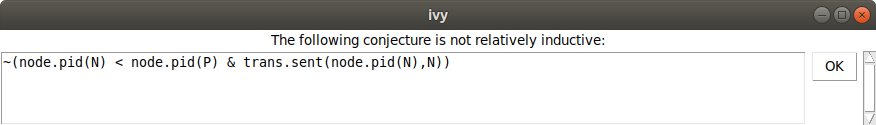And here is the counterexample to induction: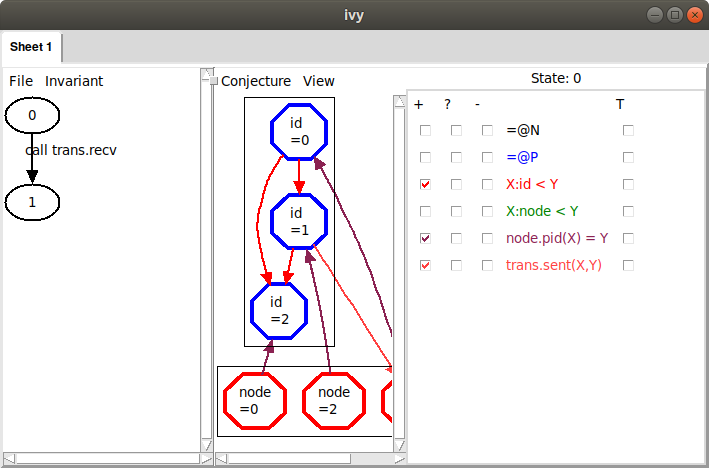Here, some externally called action transitions from state `0` satisfying our conjecture to state `1` not satisfying the conjecture. IVy is depicting state `0`, with all the relations that appear in the conjecture. It’s not immediately clear what’s wrong here, so let’s try `Invariant|Diagram` again to see what IVy thinks: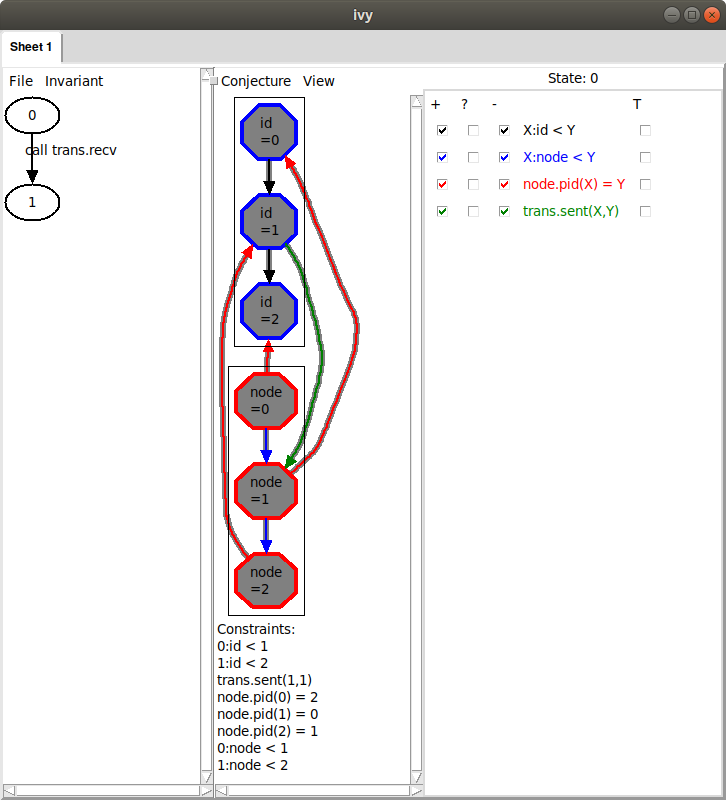Now we can see the problem: node 1’s id is arriving at node 0, but it should never have passed node 2, because node 2 has a higher id. Notice, though, that there are two additional arrows in the diagram we didn’t mention (the ones from node 0 to id 0 and id 0 to id 1). Maybe these are actually irrelevant. We could remove these manually by clicking on the corresponding facts below. However, we have another trick up our sleeve. We can use bounded checking to see if some arrows can be removed. We choose `Conjecture|Minimize` and (somewhat arbitrarily) select a bound of 4 steps. Here is what IVy says:This conjecture says that we never have nodes in the order `N < P < Q` such that `P` has a smaller id than `Q` and the id of `P` is arriving at `N`. In the graph, we see that the highlights have been removed from the two irrelevant arrows: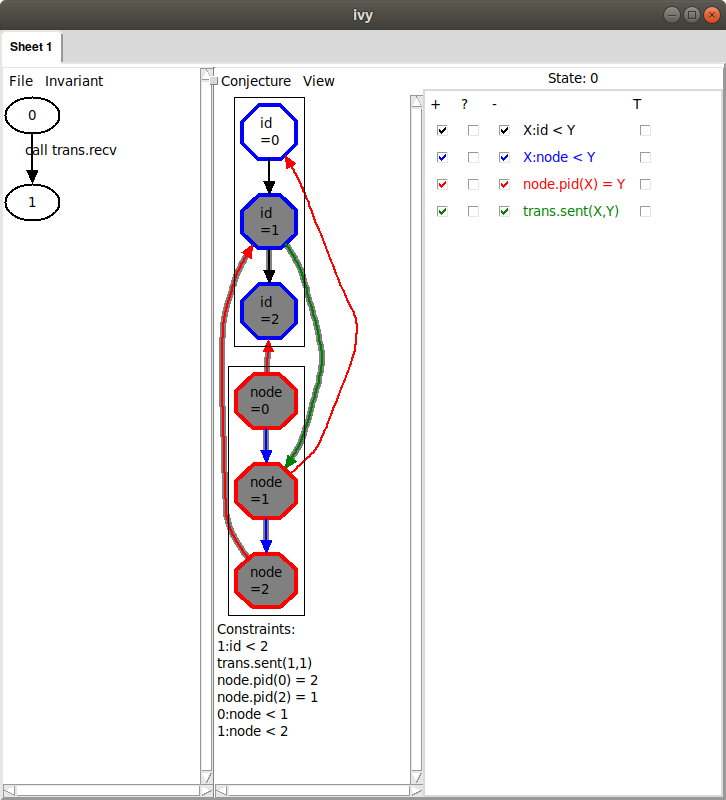IVy discovered that, within 4 steps, we can rule out the highlighted facts. By ruling out a more general situation, we obtain a stronger conjecture. Since this new conjecture seems right, let’s add it to our set by selecting `Conjecture|Strengthen`.

Now let’s try `Invariant|Check induction` again. IVy is still unhappy and says that our first conjecture is still not relatively inductive. We try `Invariant|Diagram` again and here is what we see: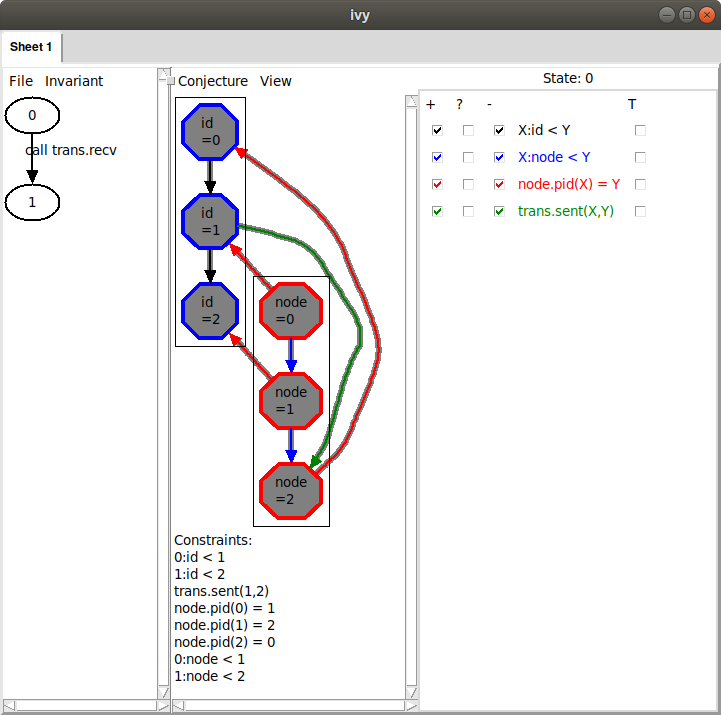This looks very similar to the diagram that led to our previous conjecture. Here, however, it’s the id of node 0 that is arriving at node 2, when it couldn’t have passed through node 1. This situation is symmetric to the previous one by rotating the ring. Unfortunately, the way we described the ring topology using a linear order has broken the ring’s rotational symmetry, so this appears as a distinct case. Later, we’ll see a way to avoid this symmetry breaking. For now, though, we’ll just slog through the cases. As before, we minimize this diagram by bounded checking. Here is the result: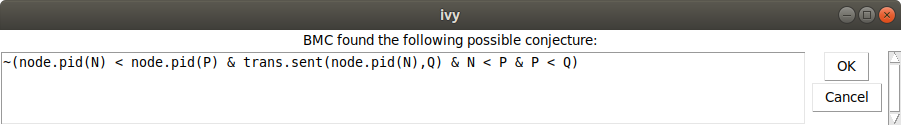IVy conjectures that we do not have nodes `N < P < Q` such that `N` has a lower id than `P` and the id of `N` is arriving at `Q`. This is just another case of the general proposition that a lower id cannot pass a higher id. We chose `Conjecture|Strengthen` to add this new conjecture to our set.

Now we try `Invariant|Check induction` (the reader may be able to guess what happens next). Again, IVy says that our first conjecture is not relatively inductive. After `Invariant|Diagram`, we see this: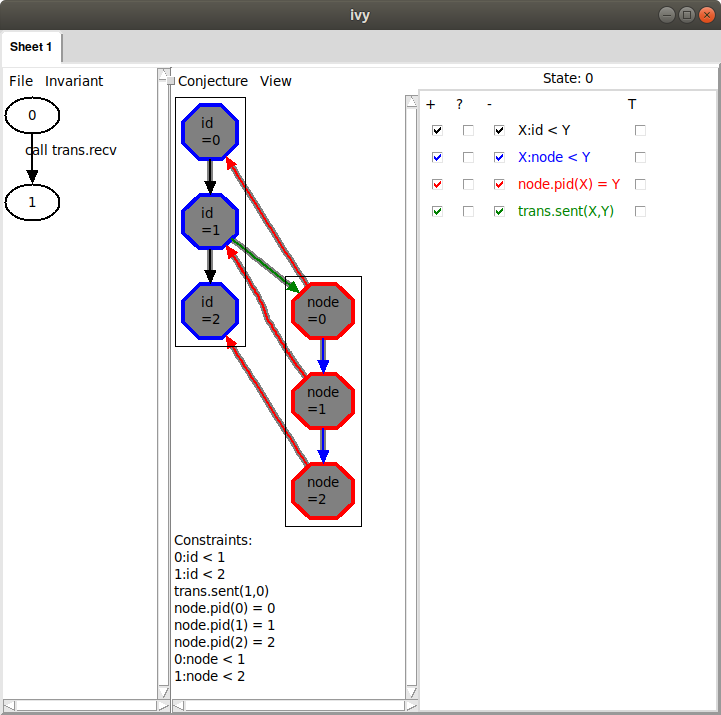This describes the same situation, where the id of node 2 is arriving at node 1. Once again, we generalize using `Conjecture|Minimize`, giving this conjecture: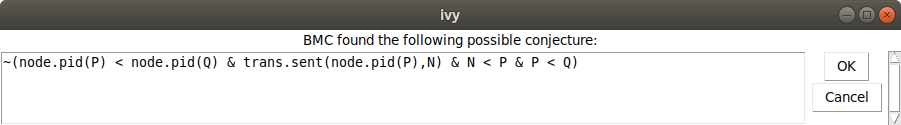We add this conjecture using `Conjecture|Strengthen`. Now when we use `Invariant|Check induction`, we get the following:That is, we have found a proof for the isolate. We can save this invariant to a file using the `File|Save invariant` command. We edit these conjectures into our implementation object `app`, like this:

``````object app = {

action async(me:node.t) = {
call trans.send(node.get_next(me),asgn.pid(me))
}

implement trans.recv(me:node.t,v:id.t) {
if v = asgn.pid(me) {       # Found a leader!
call serv.elect(me)
}
else if v > asgn.pid(me)  { # pass message to next node
call trans.send(node.get_next(me),v)
}
}

conjecture ~(asgn.pid(N) < asgn.pid(P) & trans.sent(asgn.pid(N),N))
conjecture ~(asgn.pid(P) < asgn.pid(Q) & N:node.t < P & P < Q & trans.sent(asgn.pid(P),N))
conjecture ~(asgn.pid(N) < asgn.pid(P) & N < P & P < Q & trans.sent(asgn.pid(N),Q))
conjecture ~(asgn.pid(Q) < asgn.pid(N) & N < P & P < Q & trans.sent(asgn.pid(Q),P))
}
``````

Of course, if we don’t want to mess up our pretty implementation with parts of the proof, we can put the conjectures in a separate object and include that object in the isolate definition, like this:

`````` object app_proof = {
conjecture ~(asgn.pid(N) < asgn.pid(P) & trans.sent(asgn.pid(N),N))
conjecture ~(asgn.pid(P) < asgn.pid(Q) & N:node.t < P & P < Q & trans.sent(asgn.pid(P),N))
conjecture ~(asgn.pid(N) < asgn.pid(P) & N < P & P < Q & trans.sent(asgn.pid(N),Q))
conjecture ~(asgn.pid(Q) < asgn.pid(N) & N < P & P < Q & trans.sent(asgn.pid(Q),P))
}

isolate iso_p = app with node,id,trans,serv,app_proof
``````

## How not to break the rotational symmetry

As we observed above, this proof is a bit messy because of the way we described the ring topology using a totally ordered set. There’s a different way to describe rings that avoids this problem. Here is an alternative specification of `ring_topology`:

``````module ring_topology = {
type t
relation btw(X:t,Y:t,Z:t)

property btw(W, Y, Z) & btw(W, X, Y) -> btw(X, Y, Z)
property btw(W, X, Z) & btw(X, Y, Z) -> btw(W, X, Y)
property btw(W, X, Z) & btw(X, Y, Z) -> btw(W, Y, Z)
property btw(W, Y, Z) & btw(W, X, Y) -> btw(W, X, Z)
property W = X | btw(W, X, W)
property ~btw(X, X, Y)
property ~btw(X, Y, Y)
property btw(X,Y,Z) |  Y = Z |  btw(X,Z,Y)
property btw(X,Y,Z) |  Y = X |  btw(Y,X,Z)

action get_next(x:t) returns (y:t)

object spec = {
after get_next {
assert ~btw(x,Z,y)
}
}
}
``````

Instead of putting the nodes in a total order, we define are relation `btw(X,Y,Z)` that holds when `Y` occurs on the path form `X` to `Z` in the ring. The axioms of `btw` are a bit more complex than the axioms of a total order. One the other hand, it is very easy to specify `get_next` in terms of `btw`. We say that `y` is next after `x` in the ring if there is no `Z` between `x` and `y`. You might wonder if the properties given above for `btw` are really correct. Later, when we implement `ring_topology`, we’ll prove that it has these properties.

Now, let’s try to verify the isolate `iso_app` with this new version of `ring_topology`:

``````\$ ivy ui=cti isolate=iso_app leader_election_ring_btw.ivy
``````

As before, after `Invariant|Check induction` and `Invariant|Diagram`, this is what we see: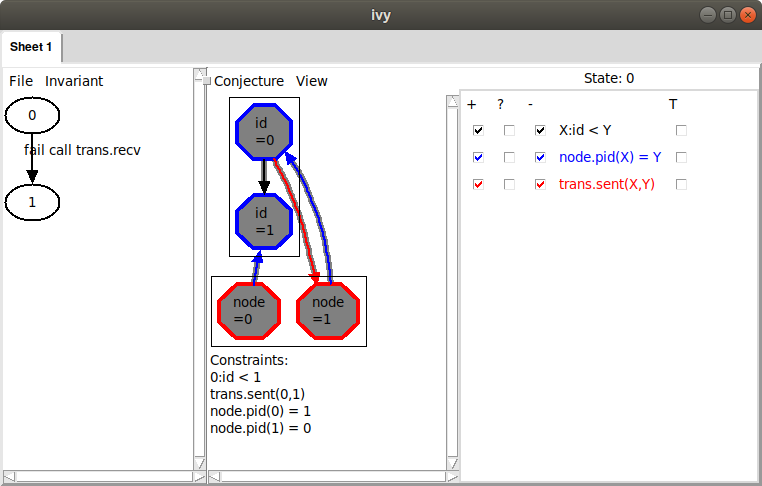That is, we have a node `1` that is receiving its own id while node `0` has a greater id. Notice, though, that on the right the relation `node.btw` doesn’t appear. This is because it is a ternary relation and IVy doesn’t know how to display it graphically in a reasonable way.

Now, as before, let’s add this as a conjecture using `Conjecture|Strengthen`. As before, `Invariant|Check induction` shows that this conjecture is not relatively inductive. Applying `Invariant|Diagram`, this is what we see: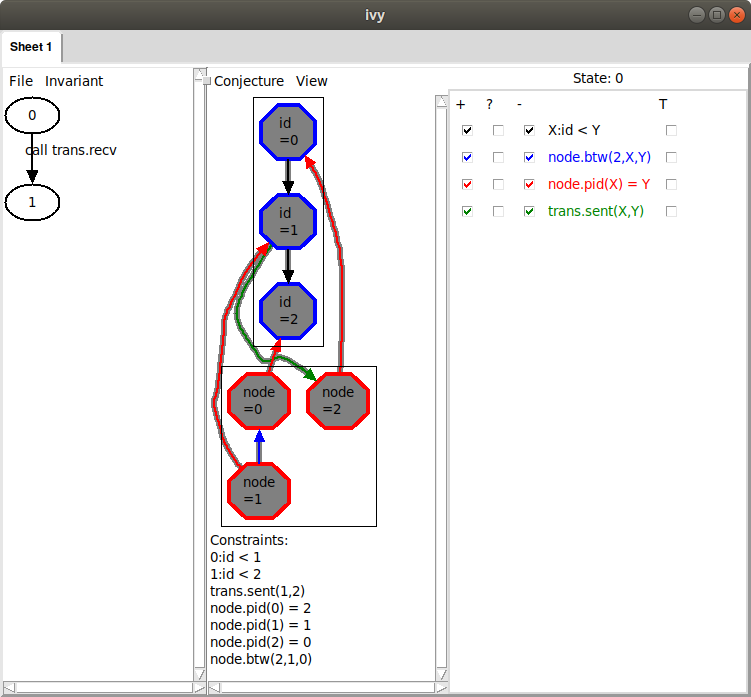This is similar to what we saw before, but notice the blue arrow from node `0` to node `1`. This corresponds to a new relation that has been added on the right: `node.btw(2,X,Y)`. From this arrow, we can infer that `node.btw(2,0,1)` holds. That is, starting at `2`, and moving around the ring, we see `0` before `1`. We can also see this fact stated below under “Constraints”. This means that, from `0`, we must pass through `1` on the way to `2`. Therefore, the id of `0` cannot possibly reach `2`, as the diagram shows.

We can try generalizing this diagram using `Conjecture|Minimize`, but in this case, there is no effect. No matter – since this looks like a reasonable conjecture, we use `Conjecture|Strengthen` to add it to our invariant. Here is the result: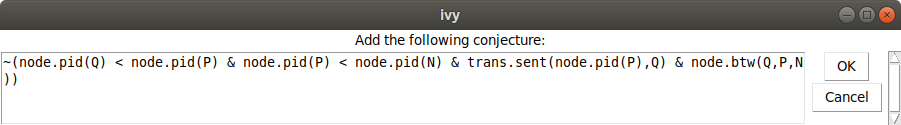This says it is not possible to have nodes `Q,N,P` in ascending order of id, such that `N` is on the way from `Q` to `P` and the id of `N` is arriving at `Q`.

Now let’s try `Invariant|Check induction`: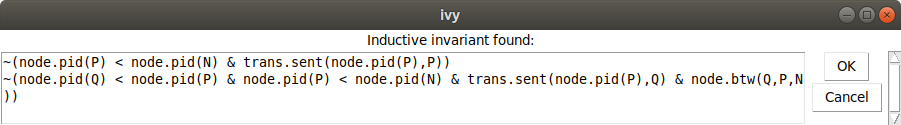Because we haven’t broken the rotational symmetry of the ring, there is just one case needed when we state the key invariant that an id can’t bypass a higher id in the ring. This illustrates that the complexity of the proof can be affected by how we write the specification. Once again, we can save this invariant and edit it into the definition of `app`.